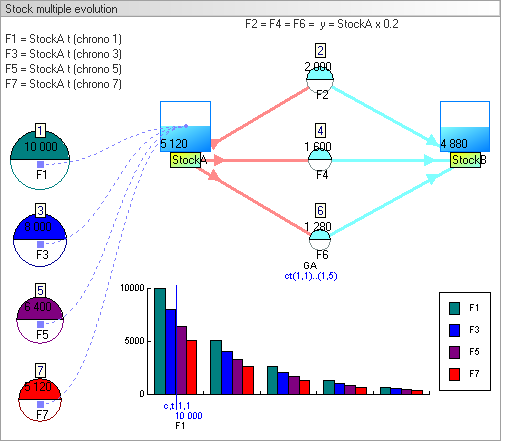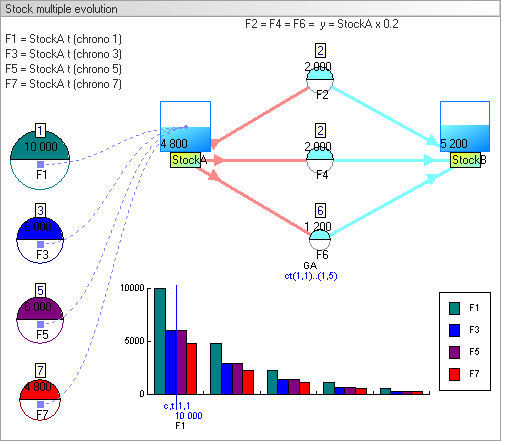Advices I wish here to give you some advices that I think are important to properly build a model. The terms of the model We must write two terms. The first terms should define : temporality of the model (example : 10 years of 12 months each) the list of variables (stocks and flows) expected results The second terms, sufficiently precise, should determine : the event type of each flow : discrete or continuous, and therefore : equation to calculate the values for each unit time, and therefore : stock-flow structure corresponding An example of insufficiently precise terms which has tree solutions : 1) calculate the quantity of water contained in a tank filled by a tap that delivers (1 x t) liters. Temporality is as follows : 1 cycle of 5 seconds, with t = 1 second. First solution : Considering that the event type is discrete; we add the quantity of water filled per unit of time : for t0, quantity (q) = 0 liter for t1, q = (0+1) = 1 liter for t2, q = (1+2) = 3 liters for t3, q = (3+3) = 6 liters for t4, q = (6+4) = 10 liters for t5, q = (10+5) = 15 liters Second solution : Considering that the event is continuous type; we calculate the surface area of the triangle whose base is the number of unit time being, and height is the corresponding number of liters : for t0, quantity (q) = 0 liter for t1, q = (1x1 / 2) = 0.5 liters for t2, q = (2x2 / 2) = 2 liters for t3, q = (3x3 / 2) = 4.5 liters for t4, q = (4x4 / 2) = 8 liters for t5, q = (5x5 / 2) = 12.5 liters Third solution : Considering that the event is continuous type; we add the quantity of water filled per unit of time : - the quantity filled per unit of time is : (t -1)+(1x1/2) => (t -1)+0.5 liters for t0, q = 0 liter for t1, q = 0 + (1-1)+0.5 = 0.5 liters for t2, q = 0.5 + (2-1)+0.5 = 2 liters for t3, q = 2 + (3-1)+0.5 = 4.5 liters for t4, q = 4.5 + (4-1)+0.5 = 8 liters for t5, q = 8 + (5-1)+0.5 = 12.5 liters Graphical representation :This terms is not precise enough because it does not specify the type of event for each flow : discrete or continuous. One might think that opening the tap is done gradually, but it could be done in stages, in fact, nothing stated in the terms. If you replace : the tank by your bank account, the tap by a bank transfer, liters by dollars One might think that the bank transfer is done in stages, i.e. all month-end, but it could be done more gradually, every day or every week, in fact, nothing stated in the terms. The temporality of the model It is important to initially define the temporality of the model and not to change it later. Indeed, many temporal parameters can be used as variables in the equations of flow. I remind you that in the software TRUE, the system time is a calendar time system and not a physical time system. The evolution of stocks between two time units In the software TRUE it is possible to several times update a stock between two units of time. I do not think this feature exists in other systems dynamics software. In this example, the stock will change tree times between two units of time : flows F2, F4 and F6 chronologies are 2,4 and 6 they are calculated and they are changing stocks A and B in that order the equation is the same for each flow : y = StockA x 0.2 flows F1, F3, F5 and F7 chronologies are 1, 3, 5 and 7 the equation is the same for each flow : y = StockA these flows are only used to measure the different values of the StockA between two time units these values are displayed in the chart Graphical representation :Same example with following change : - the flow F4 is performed at the same time as the flow F2 : flows F2 and F4 chronologies are 2 flow F6 chronology is 6 the rest of the model doesn't change but the results change Graphical representation :